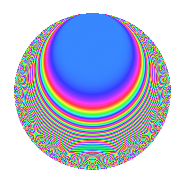# Properties

 Label 786.2.aLevel 786 Weight 2 Character orbit a Rep. character $$\chi_{786}(1,\cdot)$$ Character field $$\Q$$ Dimension 23 Newform subspaces 16 Sturm bound 264 Trace bound 7

# Related objects

## Defining parameters

 Level: $$N$$ = $$786 = 2 \cdot 3 \cdot 131$$ Weight: $$k$$ = $$2$$ Character orbit: $$[\chi]$$ = 786.a (trivial) Character field: $$\Q$$ Newform subspaces: $$16$$ Sturm bound: $$264$$ Trace bound: $$7$$ Distinguishing $$T_p$$: $$5$$, $$7$$, $$17$$

## Dimensions

The following table gives the dimensions of various subspaces of $$M_{2}(\Gamma_0(786))$$.

Total New Old
Modular forms 136 23 113
Cusp forms 129 23 106
Eisenstein series 7 0 7

The following table gives the dimensions of the cuspidal new subspaces with specified eigenvalues for the Atkin-Lehner operators and the Fricke involution.

$$2$$$$3$$$$131$$FrickeDim.
$$+$$$$+$$$$+$$$$+$$$$3$$
$$+$$$$+$$$$-$$$$-$$$$2$$
$$+$$$$-$$$$+$$$$-$$$$4$$
$$+$$$$-$$$$-$$$$+$$$$2$$
$$-$$$$+$$$$+$$$$-$$$$4$$
$$-$$$$+$$$$-$$$$+$$$$2$$
$$-$$$$-$$$$+$$$$+$$$$1$$
$$-$$$$-$$$$-$$$$-$$$$5$$
Plus space$$+$$$$8$$
Minus space$$-$$$$15$$

## Trace form

 $$23q + q^{2} + q^{3} + 23q^{4} - 2q^{5} - q^{6} - 4q^{7} + q^{8} + 23q^{9} + O(q^{10})$$ $$23q + q^{2} + q^{3} + 23q^{4} - 2q^{5} - q^{6} - 4q^{7} + q^{8} + 23q^{9} - 2q^{10} - 4q^{11} + q^{12} + 6q^{13} - 6q^{15} + 23q^{16} + 2q^{17} + q^{18} - 8q^{19} - 2q^{20} + 8q^{21} + 4q^{22} - q^{24} + 33q^{25} - 2q^{26} + q^{27} - 4q^{28} + 6q^{29} + 2q^{30} - 4q^{31} + q^{32} + 10q^{34} - 16q^{35} + 23q^{36} + 2q^{37} + 4q^{38} + 6q^{39} - 2q^{40} - 22q^{41} + 12q^{43} - 4q^{44} - 2q^{45} - 8q^{46} - 24q^{47} + q^{48} + 27q^{49} + 31q^{50} + 6q^{51} + 6q^{52} + 22q^{53} - q^{54} - 16q^{55} + 2q^{58} - 4q^{59} - 6q^{60} + 6q^{61} + 24q^{62} - 4q^{63} + 23q^{64} + 4q^{65} - 12q^{66} - 32q^{67} + 2q^{68} + 8q^{69} - 32q^{71} + q^{72} - 2q^{73} - 2q^{74} - 17q^{75} - 8q^{76} - 8q^{77} - 14q^{78} + 12q^{79} - 2q^{80} + 23q^{81} - 14q^{82} + 20q^{83} + 8q^{84} - 44q^{85} - 20q^{86} + 10q^{87} + 4q^{88} - 54q^{89} - 2q^{90} + 4q^{93} - 16q^{94} + 8q^{95} - q^{96} + 46q^{97} + 9q^{98} - 4q^{99} + O(q^{100})$$

## Decomposition of $$S_{2}^{\mathrm{new}}(\Gamma_0(786))$$ into newform subspaces

Label Dim. $$A$$ Field CM Traces A-L signs $q$-expansion
$$a_2$$ $$a_3$$ $$a_5$$ $$a_7$$ 2 3 131
786.2.a.a $$1$$ $$6.276$$ $$\Q$$ None $$-1$$ $$-1$$ $$-2$$ $$2$$ $$+$$ $$+$$ $$+$$ $$q-q^{2}-q^{3}+q^{4}-2q^{5}+q^{6}+2q^{7}+\cdots$$
786.2.a.b $$1$$ $$6.276$$ $$\Q$$ None $$-1$$ $$-1$$ $$-1$$ $$-3$$ $$+$$ $$+$$ $$-$$ $$q-q^{2}-q^{3}+q^{4}-q^{5}+q^{6}-3q^{7}+\cdots$$
786.2.a.c $$1$$ $$6.276$$ $$\Q$$ None $$-1$$ $$-1$$ $$-1$$ $$-1$$ $$+$$ $$+$$ $$+$$ $$q-q^{2}-q^{3}+q^{4}-q^{5}+q^{6}-q^{7}+\cdots$$
786.2.a.d $$1$$ $$6.276$$ $$\Q$$ None $$-1$$ $$-1$$ $$2$$ $$0$$ $$+$$ $$+$$ $$-$$ $$q-q^{2}-q^{3}+q^{4}+2q^{5}+q^{6}-q^{8}+\cdots$$
786.2.a.e $$1$$ $$6.276$$ $$\Q$$ None $$-1$$ $$-1$$ $$4$$ $$-1$$ $$+$$ $$+$$ $$+$$ $$q-q^{2}-q^{3}+q^{4}+4q^{5}+q^{6}-q^{7}+\cdots$$
786.2.a.f $$1$$ $$6.276$$ $$\Q$$ None $$-1$$ $$1$$ $$-3$$ $$5$$ $$+$$ $$-$$ $$+$$ $$q-q^{2}+q^{3}+q^{4}-3q^{5}-q^{6}+5q^{7}+\cdots$$
786.2.a.g $$1$$ $$6.276$$ $$\Q$$ None $$-1$$ $$1$$ $$-2$$ $$-2$$ $$+$$ $$-$$ $$-$$ $$q-q^{2}+q^{3}+q^{4}-2q^{5}-q^{6}-2q^{7}+\cdots$$
786.2.a.h $$1$$ $$6.276$$ $$\Q$$ None $$-1$$ $$1$$ $$1$$ $$-5$$ $$+$$ $$-$$ $$-$$ $$q-q^{2}+q^{3}+q^{4}+q^{5}-q^{6}-5q^{7}+\cdots$$
786.2.a.i $$1$$ $$6.276$$ $$\Q$$ None $$1$$ $$-1$$ $$-4$$ $$3$$ $$-$$ $$+$$ $$-$$ $$q+q^{2}-q^{3}+q^{4}-4q^{5}-q^{6}+3q^{7}+\cdots$$
786.2.a.j $$1$$ $$6.276$$ $$\Q$$ None $$1$$ $$-1$$ $$-1$$ $$-3$$ $$-$$ $$+$$ $$-$$ $$q+q^{2}-q^{3}+q^{4}-q^{5}-q^{6}-3q^{7}+\cdots$$
786.2.a.k $$1$$ $$6.276$$ $$\Q$$ None $$1$$ $$-1$$ $$4$$ $$-4$$ $$-$$ $$+$$ $$+$$ $$q+q^{2}-q^{3}+q^{4}+4q^{5}-q^{6}-4q^{7}+\cdots$$
786.2.a.l $$1$$ $$6.276$$ $$\Q$$ None $$1$$ $$1$$ $$-3$$ $$-3$$ $$-$$ $$-$$ $$+$$ $$q+q^{2}+q^{3}+q^{4}-3q^{5}+q^{6}-3q^{7}+\cdots$$
786.2.a.m $$1$$ $$6.276$$ $$\Q$$ None $$1$$ $$1$$ $$1$$ $$3$$ $$-$$ $$-$$ $$-$$ $$q+q^{2}+q^{3}+q^{4}+q^{5}+q^{6}+3q^{7}+\cdots$$
786.2.a.n $$3$$ $$6.276$$ 3.3.316.1 None $$-3$$ $$3$$ $$2$$ $$3$$ $$+$$ $$-$$ $$+$$ $$q-q^{2}+q^{3}+q^{4}+(1-\beta _{1}+\beta _{2})q^{5}+\cdots$$
786.2.a.o $$3$$ $$6.276$$ 3.3.961.1 None $$3$$ $$-3$$ $$1$$ $$1$$ $$-$$ $$+$$ $$+$$ $$q+q^{2}-q^{3}+q^{4}+\beta _{1}q^{5}-q^{6}+\beta _{1}q^{7}+\cdots$$
786.2.a.p $$4$$ $$6.276$$ 4.4.64268.1 None $$4$$ $$4$$ $$0$$ $$1$$ $$-$$ $$-$$ $$-$$ $$q+q^{2}+q^{3}+q^{4}-\beta _{3}q^{5}+q^{6}+(\beta _{1}+\cdots)q^{7}+\cdots$$

## Decomposition of $$S_{2}^{\mathrm{old}}(\Gamma_0(786))$$ into lower level spaces

$$S_{2}^{\mathrm{old}}(\Gamma_0(786)) \cong$$ $$S_{2}^{\mathrm{new}}(\Gamma_0(131))$$$$^{\oplus 4}$$$$\oplus$$$$S_{2}^{\mathrm{new}}(\Gamma_0(262))$$$$^{\oplus 2}$$$$\oplus$$$$S_{2}^{\mathrm{new}}(\Gamma_0(393))$$$$^{\oplus 2}$$